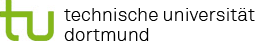You are here:

### Project P5: Integration of different omics data with regression methods

In project I5 the effect of toxicological compounds on tissues or organisms will be investigated using genomic, transcriptomic and proteomic data. As omics data in general are typically high-dimensional, the following concept is applied.

First, the different data sources will be considered and modelled separately. In these submodels, for example, it is determined which genes provide a signal with regard to the respective data type. As the targets in these different data types can be binary, continuous, or count data, the classes of Generalised Linear or Additive Regression Models (GLMs; GAMs) are useful (covering, for example, logistic, Poisson or ordinary linear regression). Depending on the dimensionality of the predictor space, different strategies can be suitable. For p<<n, conventional, unregularised regression models can be used. For determining which genes are relevant, standard significance-based approaches or subset selection approaches based on test statistics can be employed. However, if many predictors are present, multicollinearity issues become relevant and the usual stability problems of forward-backward algorithms occur, which are due to the inherent discreteness of the method (see, e.g., Breiman, 1996).

Consequently, in this situation, it is preferable to utilize suitable regularisation methods such as ridge regression (Hoerl and Kennard, 1970), lasso (Tibshirani, 1996) or boosting methods (see, e.g., Bühlmann and Hothorn, 2007). Depending on the different outcome distributions and on the different predictor structures (metric vs. categorical covariates, linear vs. nonlinear effects) the researcher has to decide which statistical modelling approach to choose. For example, if categorical predictors are to be included, instead of ordinary lasso the group lasso (Meier et al., 2008) should be used. If effect selection between linear and nonlinear effects is desired, specific boosting algorithms are suitable (see, e.g., Hothorn et al., 2010, or Groll and Tutz, 2012). For some combinations of outcome distributions and predictor structure, also methodological extensions might become necessary.

Second, all variables that provide a signal for at least one of the data types from the first stage will be included as candidates in a common model and are suitably combined. This final model is then usually based on a large number of covariates (and their interactions, if necessary), so that again the high-dimensional and non-linear regression methods from the previous paragraph need to be used or suitably extended/adjusted. Hence, this project is in close collaboration with the projects R1 and R2.

#### References

• Breiman L (1996). Heuristics of instability and stabilization in model selection. Annals of Statistics 6, 2350–83, doi: 10.1214/aos/1032181158.
• Bühlmann P, Hothorn T (2007). Boosting algorithms: Regularization, prediction and model fitting. Statistical Science 22, 477–522, doi: 10.1214/07-STS242.
• Groll A, Tutz G (2012). Regularization for generalized additive mixed models by likelihoodbased boosting. Methods of Information in Medicine 51(2), 168-177, doi: 10.3414/ME11-020021.
• Hoerl AE, Kennard RW (1970). Ridge regression: Biased estimation for nonorthogonal problems. Technometrics 12, 55–67, doi: 10.1080/00401706.1970.10488634.
• Hothorn T, Bühlmann P, Kneib T, Schmid M, Hofner B (2010). Model-based Boosting 2.0. J Machine Learning Research 11, 2109-13.
• Meier L, Van de Geer S, Bühlmann P (2008). The group lasso for logistic regression. J Royal Statistical Society B 70, 53–71, doi: 10.1111/j.1467-9868.2007.00627.x.
• Tibshirani R (1996). Regression shrinkage and selection via the lasso. J Royal Statistical Society B 58, 267–88, doi: 10.1111/j.2517-6161.1996.tb02080.x.